## 引领天下之势 全新奔驰S级郑州荣耀上市

2017年10月30日 12:22 来源：车行天下 超过：次关注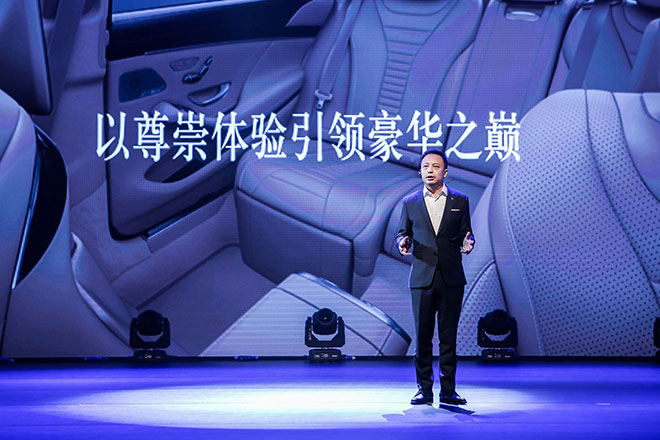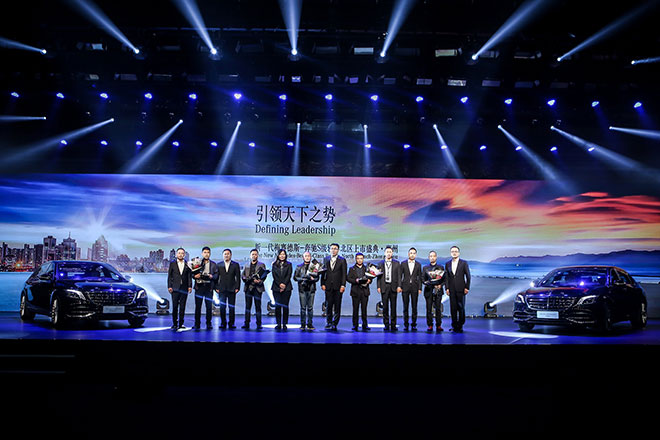•乡村道路模式同比传统近光灯更明亮、更广地照亮车辆近侧的道路边缘；

•当系统识别到正在高速公路行驶时，将启用具有更大光能输出的高速公路模式；
•转角照明灯将更多灯光分配至弯道一侧，从而改善道路照明；
•借助主动式大灯功能，大灯光束可随动转入弯道，从而提供更佳的道路照明。因此，在转动方向盘前即可照亮弯道；
•在转弯或急转弯时，转角照明灯功能可在顺畅的调光过程中启用和关闭。带环状交叉路功能的转角照明灯功能可在进入各种环状交叉路之前启用转角照明灯；
•增强型雾灯功能可更有效地照亮外侧车道，同时减少反射光对驾驶员造成的眩目；
•锁止和解锁车辆时，定位照明将通过可变换颜色的独特照明迎接和欢送驾驶员。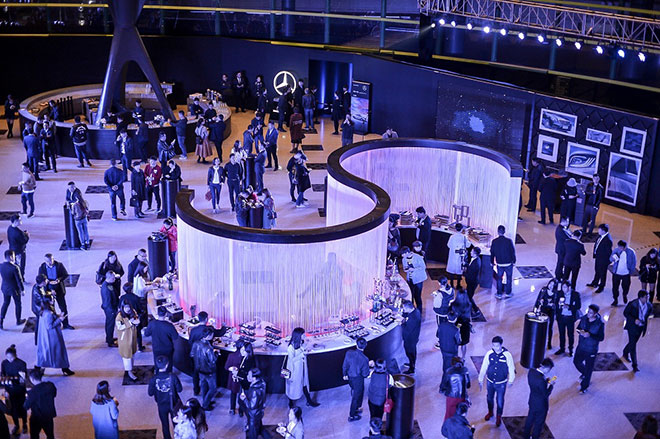•在空气动力学及声学方面进行优化的车外后视镜；

•包括大灯在内的车头前部完全密封，增强型散热器密封及气流引导实现了冷却空气的高效利用；
•冷却空气控制：带有自适应关闭功能的两件式空气隔板（AIRPANEL）用于散热器格栅的进气口及其下方保险杠的进气口，后者位于牌照框下方。根据冷却剂和空气温度等特定因素，空气隔板（AIRPANEL）控制单元向隔板执行机构发送信息。当车辆在行驶时，隔板会旋转开启几度，这样冷却空气就可通过开口。空气隔板的开启角度也将随着冷却空气需求增加而增大；
•当车速达到或超过120公里/小时之后，车身最多可降低20毫米；
•经过空气动力学优化的底盘配有庞大的护板，并一直延伸到油箱下方，这一设计也进一步减弱了路噪的影响；
•特殊的前轮扰流板；
•经过空气动力学优化的轮毂及轮胎；
•后轴上的大面积金属包裹。

“畅心醒神”功能（ENERGIZING舒适）：使驾驶员保持警觉无疑是最重要的，为实现这一目的，梅赛德斯-奔驰在这些量身定制的模式中融入了大量舒适功能。这些功能将使驾驶员在单调的驾驶状况下保持精力充沛，或有针对性地营造出舒适体验。其中包括气候控制、香氛喷雾、照明效果、音乐/视频以及座椅按摩功能。全球首创的“畅心醒神”功能（ENERGIZING舒适）将各种车载舒适系统连接起来，并带有6种融合了多种舒适功能的模式，多种功能协调设置实现舒适感和性能的提升。

“畅心醒神”功能（ENERGIZING舒适）功能一览：

•结合多种车载舒适系统，如多区域智能空调（THERMOTRONIC）、车内空气调节组件（AIR BALANCE）、环境氛围照明系统及活力按摩功能

•专为增强旅途中的驾驶体验及舒适性而倾力打造
•融入多项智能模式，可使驾驶员焕发活力、精神充沛并感到放松
•三种模式侧重点不同：借助强气流实现提神的气候控制效果、提供振奋人心的音乐或通过培训视频吸引车内乘员参与其中
•源自环境氛围照明系统的照明效果、源自车内空气调节组件（AIR BALANCE）的香氛喷雾/负离子发生器及座椅通风功能
•此外，还有3种模式可专为驾驶员营造出轻松的氛围：为实现这一目的，相应模式带有扶手加热、方向盘加热或伴随着怡人座椅加热功能的活力按摩功能

“提神”模式可在漫长的旅途中通过新鲜空气为您注入新的活力，源自多区域智能空调（THERMOTRONIC）（空气脉冲）的强大气流实现了这一惊人效果。除了香氛喷雾能够带来令人精力充沛的效果之外，车内空气调节组件（AIR BALANCE）中的负离子发生器同样扮演了一个十分重要的角色；它会给车内的驾驶员带来一种夏季暴雨过后的气候体验：纯净、新鲜。启动座椅通风功能将使得该模式的效果更加完美。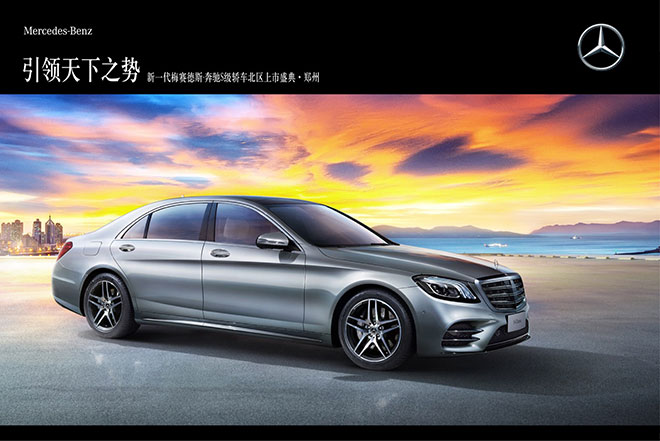•车辆长度相比上代车型增加9毫米；

•散热格栅左侧带有“MAYBACH”字样；
•大灯下方的保险杠全新结构令新型V12发动机获得更好的冷却；
•保险杠下部带有镀铬饰条及饰件，彰显了梅赛德斯-迈巴赫的非凡地位；
•远程几何多光束LED大灯（MULTIBEAM LED）独有的“三道杠”LED日间行车灯设计，令前脸颇具个性；
•后保险杠下部搭配了梅赛德斯-迈巴赫独有的镀铬饰条；
•新颖的星辰效果LED尾灯在车辆开锁及解锁时的“灯光秀”；
•新型轮毂：20英寸双5辐条轻质合金轮毂、20英寸迈巴赫20孔锻造轮毂、20英寸迈巴赫10孔锻造轮毂。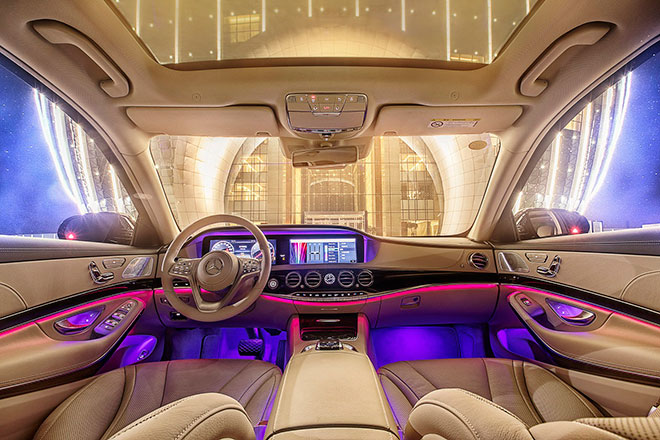S级轿车是全球首款完全使用LED光源的量产轿车。500多颗LED负责照亮路面、车外、车内及行李箱。每个大灯组中最多拥有84颗LED光源，每个尾灯最多有35颗LED光源，而车内拥有超过300颗LED光源。

100多个执行电机确保新一代S级轿车的内部舒适。包括最多21台电动辅助电机（用于实现完美的车内气候控制）、每个前排座椅最多拥有9台执行电机、每个后排座椅最多有12台执行电机。其它辅助电机包括：5台电机用于调节外后视镜，并在倒车时自动向下调整副驾后视镜以获得更清晰的车侧视野，另5台电机则用于车门和行李箱盖的自动无声关闭。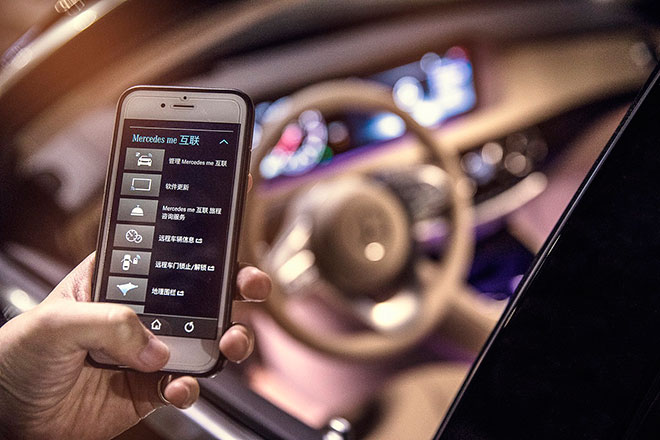#### 相关文章

﻿
• 快速找车
• 选择品牌
• 选择品牌
• A  奥迪
• A  阿斯顿·马丁
• A  阿尔法·罗密欧
• B  宝沃
• B  布加迪
• B  巴博斯
• B  保时捷
• B  宾利
• B  奔驰
• B  宝马
• B  本田
• B  别克
• B  标致
• B  比亚迪
• B  宝骏
• B  北汽制造
• B  北汽新能源
• B  北汽幻速
• B  北汽威旺
• B  北京汽车
• B  奔腾
• B  北汽绅宝
• B  北汽昌河
• C  长安欧尚
• C  长安
• C  长安凯程
• C  长城
• D  大众
• D  道奇
• D  DS
• D  东南
• D  东风风神
• D  东风风行
• D  东风小康
• D  东风风度
• D  东风
• F  福特
• F  丰田
• F  菲亚特
• F  法拉利
• F  福田
• F  福迪
• F  福汽启腾
• G  观致
• G  广汽传祺
• G  广汽吉奥
• G  GMC
• H  红旗
• H  汉腾汽车
• H  哈弗
• H  哈飞
• H  海格
• H  海马
• H  华颂
• H  黄海
• H  华泰
• H  恒天
• J  捷途
• J  几何汽车
• J  捷达
• J  吉利汽车
• J  捷豹
• J  Jeep
• J  江淮
• J  江铃
• J  金杯
• J  九龙
• J  金旅
• K  凯翼
• K  凯迪拉克
• K  克莱斯勒
• K  科尼塞克
• K  卡威
• K  开瑞
• L  路虎
• L  林肯
• L  劳斯莱斯
• L  兰博基尼
• L  雷克萨斯
• L  铃木
• L  领克
• L  雷诺
• L  理念
• L  力帆
• L  莲花汽车
• L  猎豹
• L  路特斯
• L  陆风
• M  马自达
• M  MG
• M  MINI
• M  玛莎拉蒂
• M  摩根
• M  迈凯轮
• N  纳智捷
• O  欧拉
• O  欧宝
• O  讴歌
• O  欧朗
• Q  奇瑞
• Q  起亚
• Q  启辰
• R  日产
• R  荣威
• R  瑞麒
• S  SERES赛力斯
• S  三菱
• S  斯威汽车
• S  萨博
• S  smart
• S  斯柯达
• S  斯巴鲁
• S  思铭
• S  双龙
• S  上汽大通
• S  双环
• T  特斯拉
• T  腾势
• W  蔚来
• W  沃尔沃
• W  WEY
• W  五菱汽车
• W  五十铃
• W  威兹曼
• W  威麟
• X  现代
• X  雪佛兰
• X  星途
• X  雪铁龙
• X  小鹏汽车
• X  西雅特
• Y  一汽
• Y  英菲尼迪
• Y  英致
• Y  依维柯
• Y  野马汽车
• Y  永源
• Z  众泰
• Z  中华
• Z  中兴
• Z  知豆
• 选择车系
• 选择车系
• 车型对比
• 选择品牌
• 选择品牌
• A  奥迪
• A  阿斯顿·马丁
• A  阿尔法·罗密欧
• B  宝沃
• B  布加迪
• B  巴博斯
• B  保时捷
• B  宾利
• B  奔驰
• B  宝马
• B  本田
• B  别克
• B  标致
• B  比亚迪
• B  宝骏
• B  北汽制造
• B  北汽新能源
• B  北汽幻速
• B  北汽威旺
• B  北京汽车
• B  奔腾
• B  北汽绅宝
• B  北汽昌河
• C  长安欧尚
• C  长安
• C  长安凯程
• C  长城
• D  大众
• D  道奇
• D  DS
• D  东南
• D  东风风神
• D  东风风行
• D  东风小康
• D  东风风度
• D  东风
• F  福特
• F  丰田
• F  菲亚特
• F  法拉利
• F  福田
• F  福迪
• F  福汽启腾
• G  观致
• G  广汽传祺
• G  广汽吉奥
• G  GMC
• H  红旗
• H  汉腾汽车
• H  哈弗
• H  哈飞
• H  海格
• H  海马
• H  华颂
• H  黄海
• H  华泰
• H  恒天
• J  捷途
• J  几何汽车
• J  捷达
• J  吉利汽车
• J  捷豹
• J  Jeep
• J  江淮
• J  江铃
• J  金杯
• J  九龙
• J  金旅
• K  凯翼
• K  凯迪拉克
• K  克莱斯勒
• K  科尼塞克
• K  卡威
• K  开瑞
• L  路虎
• L  林肯
• L  劳斯莱斯
• L  兰博基尼
• L  雷克萨斯
• L  铃木
• L  领克
• L  雷诺
• L  理念
• L  力帆
• L  莲花汽车
• L  猎豹
• L  路特斯
• L  陆风
• M  马自达
• M  MG
• M  MINI
• M  玛莎拉蒂
• M  摩根
• M  迈凯轮
• N  纳智捷
• O  欧拉
• O  欧宝
• O  讴歌
• O  欧朗
• Q  奇瑞
• Q  起亚
• Q  启辰
• R  日产
• R  荣威
• R  瑞麒
• S  SERES赛力斯
• S  三菱
• S  斯威汽车
• S  萨博
• S  smart
• S  斯柯达
• S  斯巴鲁
• S  思铭
• S  双龙
• S  上汽大通
• S  双环
• T  特斯拉
• T  腾势
• W  蔚来
• W  沃尔沃
• W  WEY
• W  五菱汽车
• W  五十铃
• W  威兹曼
• W  威麟
• X  现代
• X  雪佛兰
• X  星途
• X  雪铁龙
• X  小鹏汽车
• X  西雅特
• Y  一汽
• Y  英菲尼迪
• Y  英致
• Y  依维柯
• Y  野马汽车
• Y  永源
• Z  众泰
• Z  中华
• Z  中兴
• Z  知豆
• 选择车系
• 选择车系
• 选择车型
• 选择车型
• 意见反馈